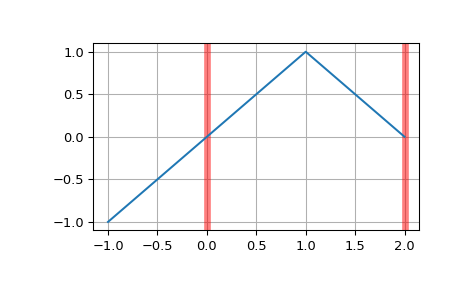# scipy.interpolate.BSpline.integrate¶

BSpline.integrate(a, b, extrapolate=None)[source]

Compute a definite integral of the spline.

Parameters: a : float Lower limit of integration. b : float Upper limit of integration. extrapolate : bool, optional whether to extrapolate beyond the base interval, t[k] .. t[-k-1], or take the spline to be zero outside of the base interval. Default is True. I : array_like Definite integral of the spline over the interval [a, b].

Examples

Construct the linear spline x if x < 1 else 2 - x on the base interval $$[0, 2]$$, and integrate it

>>> from scipy.interpolate import BSpline
>>> b = BSpline.basis_element([0, 1, 2])
>>> b.integrate(0, 1)
array(0.5)


If the integration limits are outside of the base interval, the result is controlled by the extrapolate parameter

>>> b.integrate(-1, 1)
array(0.0)
>>> b.integrate(-1, 1, extrapolate=False)
array(0.5)

>>> import matplotlib.pyplot as plt
>>> fig, ax = plt.subplots()
>>> ax.grid(True)
>>> ax.axvline(0, c='r', lw=5, alpha=0.5)  # base interval
>>> ax.axvline(2, c='r', lw=5, alpha=0.5)
>>> xx = [-1, 1, 2]
>>> ax.plot(xx, b(xx))
>>> plt.show()